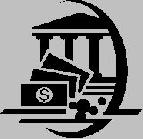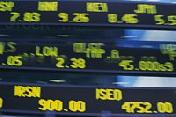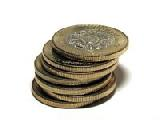Financial Terms Indicated yield

# Definition of Indicated yield## Indicated yield

The yield, based on the most recent quarterly rate times four. To determine the yield, divide
the annual dividend by the price of the stock. The resulting number is represented as a percentage. See:
dividend yield.

# Related Terms:

## Dividend yield (Funds)

indicated yield represents return on a share of a mutual fund held over the past 12
months. Assumes fund was purchased 1 year ago. Reflects effect of sales charges (at current rates), but not
redemption charges.

## Dividend yield (Stocks)

indicated yield represents annual dividends divided by current stock price.

## Annual percentage yield (APY)

The effective, or true, annual rate of return. The APY is the rate actually
earned or paid in one year, taking into account the affect of compounding. The APY is calculated by taking
one plus the periodic rate and raising it to the number of periods in a year. For example, a 1% per month rate
has an APY of 12.68% (1.01^12).

## Bond equivalent yield

Bond yield calculated on an annual percentage rate method. Differs from annual
effective yield.

## Bond-equivalent yield

The annualized yield to maturity computed by doubling the semiannual yield.

## Capital gains yield

The price change portion of a stock's return.

## Convenience yield

The extra advantage that firms derive from holding the commodity rather than the future.## Coupon equivalent yield

True interest cost expressed on the basis of a 365-day year.

## Current yield

For bonds or notes, the coupon rate divided by the market price of the bond.

## Earnings yield

The ratio of earnings per share after allowing for tax and interest payments on fixed interest
debt, to the current share price. The inverse of the price/earnings ratio. It's the Total Twelve Months earnings
divided by number of outstanding shares, divided by the recent price, multiplied by 100. The end result is
shown in percentage.

## Effective annual yield

Annualized interest rate on a security computed using compound interest techniques.

## Equivalent bond yield

Annual yield on a short-term, non-interest bearing security calculated so as to be
comparable to yields quoted on coupon securities.

## Equivalent taxable yield

The yield that must be offered on a taxable bond issue to give the same after-tax
yield as a tax-exempt issue.

## Flattening of the yield curve

A change in the yield curve where the spread between the yield on a long-term
and short-term Treasury has decreased. Compare steepening of the yield curve and butterfly shift.

See:junk bond.

## Indicated dividend

Total amount of dividends that would be paid on a share of stock over the next 12 months
if each dividend were the same amount as the most recent dividend. Usually represent by the letter "e" in
stock tables.## Liquid yield option note (LYON)

Zero-coupon, callable, putable, convertible bond invented by Merrill

## Liquid yield option note (LYON)

Zero-coupon, callable, putable, convertible bond invented by Merrill Lynch & Co.

## Non-parallel shift in the yield curve

A shift in the yield curve in which yields do not change by the same
number of basis points for every maturity. Related: Parallel shift in the yield curve.

## Parallel shift in the yield curve

A shift in the yield curve in which the change in the yield on all maturities is
the same number of basis points. In other words, if the 3 month T-bill increases 100 basis points (one
percent), then the 6 month, 1 year, 5 year, 10 year, 20 year, and 30 year rates increase by 100 basis points as
well.
Related: Non-parallel shift in the yield curve.

## Pure yield pickup swap

Moving to higher yield bonds.

## Realized compound yield

yield assuming that coupon payments are invested at the going market interest
rate at the time of their receipt and rolled over until the bond matures.

The ratio of the yield spread to the yield level.

## Reoffering yield

In a purchase and sale, the yield to maturity at which the underwriter offers to sell the bonds
to investors.

## Required yield

Generally referring to bonds, the yield required by the marketplace to match available returns
for financial instruments with comparable risk.

## Riding the yield curve

Buying long-term bonds in anticipation of capital gains as yields fall with the
declining maturity of the bonds.

## Steepening of the yield curve

A change in the yield curve where the spread between the yield on a long-term
and short-term Treasury has increased. Compare flattening of the yield curve and butterfly shift.## Weighted average portfolio yield

The weighted average of the yield of all the bonds in a portfolio.

## Yield

The percentage rate of return paid on a stock in the form of dividends, or the effective rate of interest
paid on a bond or note.

## Yield curve

The graphical depiction of the relationship between the yield on bonds of the same credit quality
but different maturities. Related: Term structure of interest rates. Harvey (1991) finds that the inversions of
the yield curve (short-term rates greater than long term rates) have preceded the last five U.S. recessions. The
yield curve can accurately forecast the turning points of the business cycle.

## Yield curve option-pricing models

Models that can incorporate different volatility assumptions along the
yield curve, such as the Black-Derman-Toy model. Also called arbitrage-free option-pricing models.

## Yield curve strategies

Positioning a portfolio to capitalize on expected changes in the shape of the Treasury yield curve.

## Yield ratio

The quotient of two bond yields.

Strategies that involve positioning a portfolio to capitalize on expected changes in
yield spreads between sectors of the bond market.

## Yield to call

The percentage rate of a bond or note, if you were to buy and hold the security until the call date.
This yield is valid only if the security is called prior to maturity. Generally bonds are callable over several
years and normally are called at a slight premium. The calculation of yield to call is based on the coupon rate,
length of time to the call and the market price.

## Yield to maturity

The percentage rate of return paid on a bond, note or other fixed income security if you
buy and hold it to its maturity date. The calculation for YTM is based on the coupon rate, length of time to
maturity and market price. It assumes that coupon interest paid over the life of the bond will be reinvested at
the same rate.

## Yield to worst

The bond yield computed by using the lower of either the yield to maturity or the yield to call
on every possible call date.

## dividend yield ratio

Cash dividends paid by a business over the most
recent 12 months (called the trailing 12 months) divided by the current
market price per share of the stock. This ratio is reported in the daily
stock trading tables in the Wall Street Journal and other major newspapers.

## Bond Equivalent Yield

Bond yield calculated on an annual percentage rate method

## Effective Annual Yield

Annualized rate of return on a security computed using compound
interest techniques

## Yield Curve

A graphical representation of the level of interest rates for
securities of differing maturities at a specific point of time

## Yield to Maturity

The measure of the average rate of return that will be earned on a
debt security held until it matures

## labor yield variance

(standard mix X actual hours X standard rate) - (standard mix X standard hours X standard rate);
it shows the monetary impact of using more or fewer total hours than the standard allowed

## material yield variance

(standard mix X actual quantity X standard price) - (standard mix X standard quantity X standard price);
it computes the difference between the
actual total quantity of input and the standard total quantity
allowed based on output and uses standard mix and
standard prices to determine variance

## process quality yield

the proportion of good units that resulted from the activities expended

## yield

the quantity of output that results from a specified input

## yield ratio

the expected or actual relationship between input and output

## Par yield curve

The yield curve of bonds selling at par, or face, value.

See Zero curve.

## Yield

a. Measure of return on an investment, stated as a percentage of price.
yield can be computed by dividing return by purchase price, current market
value, or other measure of value.
b. Income from a bond expressed as an
annualized percentage rate.
c. The nominal annual interest rate that gives a
future value of the purchase price equal to the redemption value of the security.
Any coupon payments determine part of that yield.

## Yield curve

Graph of yields (vertical axis) of a particular type of security
versus the time to maturity (horizontal axis). This curve usually slopes
securities that have a longer time to maturity. The benchmark yield curve is
for U.S. Treasury securities with maturities ranging from three months to 30
years. See Term structure.

## Yield to maturity

A measure of the average rate of return that will be earned
on a bond if held to maturity.

## Zero curve, zero-coupon yield curve

A yield curve for zero-coupon bonds;
zero rates versus maturity dates. Since the maturity and duration (Macaulay
duration) are identical for zeros, the zero curve is a pure depiction of supply/
demand conditions for loanable funds across a continuum of durations and
maturities. Also known as spot curve or spot yield curve.

## Production yield variance

The difference between the actual and budgeted proportions
of product resulting from a production process, multiplied by the standard unit cost.

## current yield

Annual coupon payments divided by bond price.

## yield curve

Graph of the relationship between time to maturity and yield to maturity.

## yield to maturity

Interest rate for which the present value of the bond’s payments equals the price.

## Current Yield

The percentage return on a financial asset based on the current price of the asset, without reference to any expected change in the price of the asset. This contrasts with yield-to-maturity, for which the calculation includes expected price changes. See also yield.

## Yield

The interest rate that makes the present value of a stream of future payments associated with an asset equal to the current price of that asset. Also called yield to maturity. See also current yield.

## Yield curve

A graph showing how the yield on bonds varies with time to maturity.